• 北京54坐标转WGS84坐标
2022-01-15 19:00:08

# 一、PROJ4JS是什么？

PROJ4JS用于将坐标从一个坐标系转换到另一个坐标系的 JavaScript 库，包括基准面转换。网址：PROJ4JS官网

# 二、使用步骤

## 1.安装proj4

在项目路径下安装，proj4官网介绍三种安装方式，本文只采用一种。

npm install proj4


## 2.引入库

代码如下（示例）：

import proj4 from 'proj4';
//'proj4'为安装后文件所在位置


## 2.坐标转换

代码如下（示例）：

//将经纬度2437(北京54)的坐标系转换成4326(WGS84)
var fromProjection = "+proj=tmerc +lat_0=0 +lon_0=120 +k=1 +x_0=500000 +y_0=0 +ellps=krass +towgs84=15.8,-154.4,-82.3,0,0,0,0 +units=m +no_defs" ;
var toProjection ="+proj=longlat +ellps=WGS84 +datum=WGS84 +no_defs";
var transformPoint = proj4(fromProjection,toProjection,[479083,5381250,648]);//[y,x,z]
console.log(transformPoint);


# 总结

在安装proj4库时使用npm install proj4无法安装成功，后来使用cnpm install proj4才完成安装。vue.js 前端
更多相关内容
• 支持GPS WGS84 国家2000 西安80 北京54坐标系的大地、经纬度坐标和平面坐标之间的转换，换带计算，不同坐标系之间的7参数、4参数转换 注意：运行时，杀毒软件可能会提示存在木马，这是授权机制引起的，请忽略，或者...
• 用于北京1954坐标系转换参数，从arc中整理出来，放心使用
• 本工具为excel进行开发，针对北京54与wgs84坐标系的相互转换功能。精准
• 结合原有北京54坐标系和由GPS成果换算得的54坐标系之间进行相互转换的实例，探讨了公共点的误差对转换模型的影响，提出了转换计算前应对公共点进行筛选的设想，并利用Baarda数据探测法原理，对公共点进行筛选，剔除...
• 自己写的wgs84坐标转成北京54坐标，java源码，本人已经测试过，能用，首先要找一个坐标软件求得七参（随便一个坐标软件都行），然后再用此源码进行坐标转换，
• 北京54坐标正算，通过经纬度计算平面XY坐标北京54坐标正算，通过经纬度计算平面XY坐标
• 正射影像坐标国家2000转北京54坐标。超清详细视频
• 北京54坐标系与西安80坐标坐标转换公式与算法
• 天津90坐标北京54坐标转换公式，方便天津附近测绘服务。
• 基于开源的proj4实现的wgs84到北京54坐标转换，适用于x86平台，包换源码及开源库。
• 主要介绍了运用Excel表格完成GPS测定结果的WGS-84坐标系与北京1954坐标系的坐标变换方法,证明使用Excel软件进行GPS坐标转换、高斯投影等数据处理是可行的,也是高效的,可供同行参考。
• WGS84 CGCS2000 北京54 西安80 大地坐标精确转换工具。含计算工具和实例文本，坐标转换主要应用在工程测量以及GIS相关的很多领域。不用的领域对其转换的精度要求也不一样，在使用GOOLE EARTH时或者GIS地图中，精度在...
• 1954年北京坐标系可以认为是前苏联1942年坐标系的延伸。它的原点不在北京而是在前苏联的普尔科沃。 北京54坐标系特点： a．属参心大地坐标系； b．采用克拉索夫斯基椭球的两个几何参数； c....

### 北京54坐标系：

北京54坐标系(BJZ54)是指北京54坐标系为参心大地坐标系，大地上的一点可用经度L54、纬度M54和大地高H54定位，它是以克拉索夫斯基椭球为基础，经局部平差后产生的坐标系。1954年北京坐标系可以认为是前苏联1942年坐标系的延伸。它的原点不在北京而是在前苏联的普尔科沃。

### 北京54坐标系特点：

a．属参心大地坐标系

b．采用克拉索夫斯基椭球的两个几何参数；

c.大地原点在原苏联的普尔科沃；

d．采用多点定位法进行椭球定位

e．高程基准为 1954年青岛验潮站求出的黄海平均海水面

f．高程异常以原苏联 1955年大地水准面重新平差结果为起算数据。按我国天文水准路线推算而得。

### 参数：

椭球坐标参数如下：

长半轴a=6378245m；

短半轴=6356863.0188m；

扁率α=1/298.3；

第一偏心率平方 =0.006693421622。

### 西安80坐标系：

1978年4月在西安召开全国天文大地网平差会议，确定重新定位，建立我国新的坐标系。为此有了1980年国家大地坐标系。1980年国家大地坐标系采用地球椭球基本参数为1975年国际大地测量与地球物理联合会第十六届大会推荐的数据，即IAG75地球椭球体。该坐标系的大地原点设在我国中部的陕西省泾阳县永乐镇，位于西安市西北方向约60公里。

### 西安80坐标系特点：

西安80坐标系的主要优点在于：克氏椭球只给定了长半轴与扁率，仅描述了地球面的几何形状，而西安80坐标系的椭球体参数精度更高，4个参数是一个完整的系统；定位采用的椭球体面与我国大地水准面符合较好；天文大地坐标网传算误差和天文重力水准路线传算误差都不太大；天文大地坐标网的坐标经过了全国性整体平差，坐标统一，精度优良，可以满足1：5000甚至更大比例尺测图的要求等。

### 参数：

长半轴a=6378140±5（m）

短半轴b=6356755.2882（m）

扁 率α=1/298.257

第一偏心率平方 =0.00669438499959 第二偏心率平方=0.00673950181947

ARCGIS定义：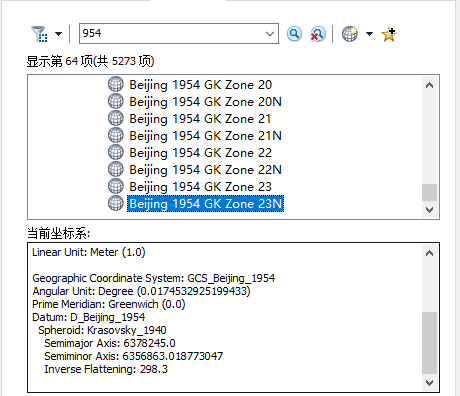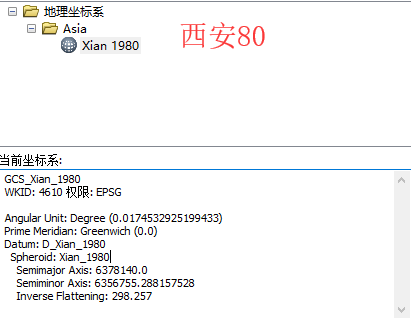### 北京54坐标系与西安80坐标系的转换：

北京54坐标系和西安80坐标系之间的转换其实是两种不同的椭球参数之间的转换，一般而言比较严密的是用七参数布尔莎模型，即X平移，Y平移，Z平移，X旋转（WX），Y旋转（WY），Z旋转（WY），尺度变化（DM）。若得七参数就需要在一个地区提供3个以上的公共点坐标对（即北京54坐标下x、y、z和西安80坐标系下x、y、z），可以向地方测绘局获取。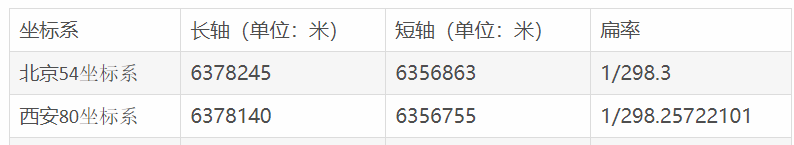### 详细的转换原理及代码。后续更新。。。。。

展开全文• 北京54坐标系与西安80坐标坐标转换公式与算法.doc
• 实现大地坐标与空间直角坐标和平面坐标的相互转换以及不同参考椭球的坐标系转换，内含84到54坐标转换示例！
• //第五步将54平面坐标X54、Y54换算到北京地方坐标Xdf、Ydf double dx = (X54 - 4414702.66) / 1000; double dy = (Y54 - 500000) / 1000; double spdx = 0; double spdy = 0; if (iType == 7){ ...
package com.towery.smoke.data;

import java.text.DecimalFormat;
import java.util.HashMap;
import java.util.Map;

public class GpsToBj {

public static void main(String[] args) {
Map<String, String> gpStoBJ = GPStoBJ("39.9084152661928","116.502275001177");
String gpsx = gpStoBJ.get("bjx");
String gpsy = gpStoBJ.get("bjy");
System.out.println("gpsx="+gpsx+",gpsy="+gpsy);
}

/**
* GPS TO BJ
* @param pointx
* @param pointy
* @return
*/
public static Map<String,String> GPStoBJ(String pointx,String pointy){
Map<String,String> map = new HashMap<String, String>();
try {
DecimalFormat df = new DecimalFormat("#.##");
double L0 = 117;
double h = 150;
double L = Double.parseDouble(pointy);//经度
double B = Double.parseDouble(pointx);;//纬度
double[] bj = WGS842BJ(L, B, L0, h);
String pointxsde = df.format(bj);
String pointysde = df.format(bj);
map.put("bjx", pointxsde);
map.put("bjy", pointysde);
} catch (Exception e) {
e.printStackTrace();
}
return map;
}
/**
* WGS84与BJ54相互转换
* gps to bj
* @param L
* @param B
* @param L0
* @param h
* @return
*/
public static double[] WGS842BJ(double L, double B, double L0, double h){
double[] result = new double;

//第一步将WGS84的LB转换为WGS84的空间直角坐标KX84、KY84、KZ84
double Ta = 6378137; //6378245 //--椭球体长半轴-54=6378245;WGS84=6378137
double Tb = 6356752.3142; //6356863.0188 //--椭球体短半轴-54=6356863.0188;WGS84=6356752.3142
B = deg(B);
L0 = deg(L0);
L = deg(L);
double E = Math.pow((1 - Math.pow(Tb / Ta, 2)), 0.5); //-- 第一偏心率

double N = Ta / ( Math.pow(1 - E * E * Math.sin(B) * Math.sin(B), 0.5)); // '--卯酉圈曲率半径

double KX84 = (N + h) * Math.cos(B) * Math.cos(L);
double KY84 = (N + h) * Math.cos(B) * Math.sin(L);
double KZ84 = (N * (1 - E * E) + h) * Math.sin(B);

int iType = 7;

double X0 = 0.0;	//平移X0
double Y0 = 0.0;	//平移Y0
double Z0 = 0.0;	//平移Z0
double tx = 0.0;	//X轴旋转角度TX
double ty = 0.0;	//Y轴旋转角度TY
double tz = 0.0;	//Z轴旋转角度TZ
double k = 0.0;		//缩放尺度K

if (iType == 7){
//第二步将WGS84空间直角坐标通过七参数转换为54空间直角坐标KX54、KY54、KZ54
X0 = 123.072;	//平移X0
Y0 = 279.5004;	//平移Y0
Z0 = 27.0206;	//平移Z0
tx = -0.000959959166667;	//X轴旋转角度TX
tx = deg(tx);	//调用子过程将度换算到弧度
ty = 0.000614433888889;	//Y轴旋转角度TY
ty = deg(ty);	//调用子过程将度换算到弧度
tz = -0.001131175277778;	//Z轴旋转角度TZ
tz = deg(tz);	//调用子过程将度换算到弧度
k = -0.000014693;	//缩放尺度K
} else {
//第二组三参数
X0 = -36.398;	//平移X0
Y0 = 73.535;	//平移Y0
Z0 = 71.968;	//平移Z0
tx = 0;			//X轴旋转角度TX
tx = deg(tx);	//调用子过程将度换算到弧度
ty = 0;			//Y轴旋转角度TY
ty = deg(ty);	//调用子过程将度换算到弧度
tz = 0;			//Z轴旋转角度TZ
tz = deg(tz);	//调用子过程将度换算到弧度
k = 0;			//缩放尺度K
}

double KX54 = X0 + (1 + k) * (1 * KX84 + tz * KY84 - ty * KZ84);
double KY54 = Y0 + (1 + k) * (-tz * KX84 + 1 * KY84 + tx * KZ84);
double KZ54 = Z0 + (1 + k) * (ty * KX84 - tx * KY84 + 1 * KZ84);

//第三步将54空间直角坐标反算到54大地坐标B54、L54

Ta = 6378245;	// '--椭球体长半轴-54=6378245;WGS84=6378137
Tb = 6356863.0188;	// '--椭球体短半轴-54=6356863.0188;WGS84=6356752.3142
E = Math.pow(1 - Math.pow(Tb / Ta, 2), 0.5);// '-- 第一偏心率

double L54 = Math.atan(KY54 / KX54);

double tt = KZ54 * E * E;
double sinB = (KZ54 + tt) / Math.pow(KX54 * KX54 + KY54 * KY54 + Math.pow(KZ54 + tt, 2), 0.5);
int myNum = 1;
double deltsinB = 1;

while (deltsinB > 0.0000001 || myNum <= 10){
myNum = myNum + 1;
double sinB0 = sinB;
N = Ta / (Math.pow(1 - E * E * sinB * sinB,0.5));//  '--卯酉圈曲率半径
tt = N * E * E * sinB;
sinB = (KZ54 + tt) / Math.pow(KX54 * KX54 + KY54 * KY54 + Math.pow(KZ54 + tt,2),0.5);
deltsinB = Math.abs(sinB - sinB0);
}

double B54 = Math.asin(sinB);
N = Ta / (Math.pow(1 - E * E * sinB * sinB, 0.5));

L54 = L54 * 180 / 3.1415926536;
B54 = B54 * 180 / 3.1415926536;

if (L54 < 0){
L54 = 180 + L54;
}
if (B54 < 0){
B54 = 180 + B54;
}

//第四步将54大地坐标B54、L54进行高斯投影正算得到54平面坐标X54、Y54

//调用子过程将度分秒换算到弧度
L54 = deg(L54);
B54 = deg(B54);

Ta = 6378245;// ' 6378137'--椭球体长半轴-54=6378245;WGS84=6378137
Tb = 6356863.0188;// '6356752.3142  '--椭球体短半轴-54=6356863.0188;WGS84=6356752.3142
E = Math.pow(1 - Math.pow(Tb / Ta, 2),0.5);// '-- 第一偏心率
double e1 = Math.pow(Math.pow(Ta / Tb, 2) - 1, 0.5);// ' -- 第二偏心率
N = (Ta * Ta / Tb) / (1 + e1 * e1 * Math.cos(B54) * Math.cos(B54));// - -卯酉圈曲率半径

double t = Math.tan(B54) * Math.tan(B54);
double C = e1 * e1 * Math.cos(B54) * Math.cos(B54);
double AA = (L54 - L0) * Math.cos(B54);
double M = Ta * ((1 - Math.pow(E, 2) / 4 - 3 * Math.pow(E,4) / 64 - 5 * Math.pow(E,6) / 256) * B54 - (3 * Math.pow(E,2) / 8 + 3 * Math.pow(E,4) / 32 + 45 * Math.pow(E,6) / 1024) * Math.sin(2 * B54) + (15 * Math.pow(E,4) / 256 + 45 * Math.pow(E,6) / 1024) * Math.sin(4 * B54) - (35 * Math.pow(E,6) / 3072) * Math.sin(6 * B54));
N = Ta / Math.pow(1 - E * E * Math.sin(B54) * Math.sin(B54), 0.5);// '= (Ta * Ta / Tb) / (1 + e1 * e1 * Cos(B54) * Cos(B54)) ^ 0.5
double FE = 500000;

double X54 = M + N * Math.tan(B54) * (AA * AA / 2 + (5 - t + 9 * C + 4 * C * C) * Math.pow(AA, 4) / 24) + (61 - 58 * t + t * t + 270 * C - 330 * t * C) * Math.pow(AA, 6) / 720;
double Y54 = FE + N * (AA + (1 - t + C) * Math.pow(AA, 3) / 6 + (5 - 18 * t + t * t + 14 * C - 58 * t * C) * Math.pow(AA, 5) / 120);

//第五步将54平面坐标X54、Y54换算到北京地方坐标Xdf、Ydf
double dx = (X54 - 4414702.66) / 1000;
double dy = (Y54 - 500000) / 1000;

double spdx = 0;
double spdy = 0;

if (iType == 7){
spdx = 7.345806056;
spdy = 15.48276601;
}

double Xdf = 300202.06 + 1000.01161 * dx + 7.269 * dy - 9.98 * 0.000001 * (dx * dx - dy * dy) + 1.37 * 0.001 * dx * dy + spdx;
double Ydf = 555594.14 - 7.269 * dx + 1000.01161 * dy - 6.84 * 0.0001 * (dx * dx - dy * dy) - 2 * 0.000001 * dx * dy + spdy;

result = Xdf;
result = Ydf;
//result = X54;
//result = Y54;

return result;
}

public static double deg(double a){
double result = a;
//输入的角度是以度为单位的，XXX.XXXXXXX度

double k = Math.signum(a);
result = Math.abs(a);
result = result * Math.PI / 180;
result = result * k;

return result;
}
}


展开全文java
• 采用正算原始公式实现的高斯投影北京54坐标系统的坐标转换函数，适合GPS定位时使用
• C#编写的，在WGS84，西安80，北京54不同椭球体参数下的高斯正反算坐标转换源代码
• 自定义 坐标系（ 坐标系（ 坐标系（ 北京 54 、西安 80 、2000 坐标系 ）
• 北京54坐标系向国家2000大地坐标系的转换.doc文档
• 北京54坐标系(BJZ54)是指北京54坐标系为参心大地坐标系，大地上的一点可用经度L54、纬度M54和大地高H54定位，它是以克拉索夫斯基椭球为基础，经局部平差后产生的坐标系。 2.坐标历史 新中国成立以后，全国范围内...

1. 概述

北京54坐标系(BJZ54)是指北京54坐标系为参心大地坐标系，大地上的一点可用经度L54、纬度M54和大地高H54定位，它是以克拉索夫斯基椭球为基础，经局部平差后产生的坐标系。

2. 坐标历史

新中国成立以后，全国范围内开展了正规的、全面的大地测量和测图工作，迫切需要建立一个参心大地坐标系。由于当时政治环境是"一边倒"地亲近苏联，所以采用了前苏联的克拉索夫斯基椭球参数，并与前苏联1942年坐标系进行联测，通过计算建立了我国大地坐标系。于1954年完成测定工作，故命名为"1954年北京坐标系"。 因此，1954年北京坐标系可以认为是前苏联1942年坐标系的延伸。它的大地原点不在北京，而是在前苏联的普尔科沃（Pulkovo）。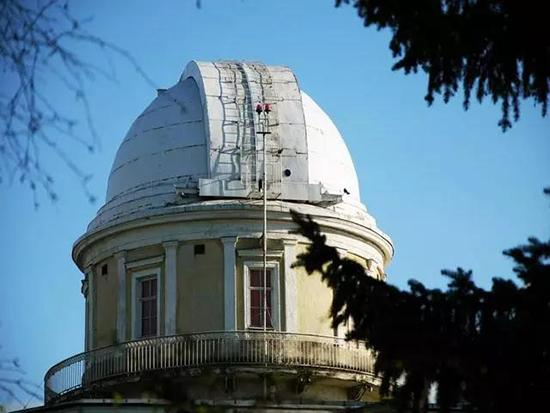普尔科沃天文台

3. 椭球参数

椭球坐标参数如下：

长半轴a=6378245m；

短半轴=6356863.0188m；

扁率α=1/298.3；

第一偏心率平方 =0.006693421622。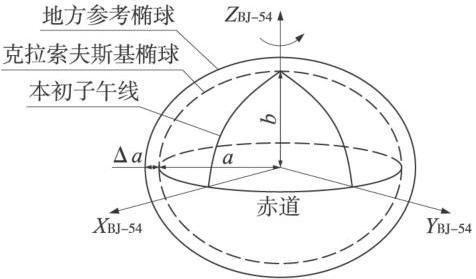北京54椭球

4. 坐标特点

①属于参心大地坐标系；

②采用克拉索夫斯基椭球的两个几何参数；

③大地原点在原苏联的普尔科沃；

④采用多点定位法进行椭球定位；

⑤高程基准为1954年青岛验潮站求出的黄海平均海水面；

⑥高程异常以原苏联 1955年大地水准面重新平差结果为起算数据。按我国天文水准路线推算而得。1957年鄂尔多斯航测像片判读

5. 坐标局限性

在当时，北京54坐标系满足了我国测绘事业发展的急需，此后很长一段时间内，也为国家经济建设做出了应有的贡献。但是随着测绘新理论、新技术的不断发展，北京54坐标系的缺点也愈加明显。最大的问题就是精度不够、误差较大。原因是北京54坐标系所采用的克拉索夫斯基椭球参数误差较大，与现代精确值相比长半轴大了约109m。并且，参考椭球面与我国似大地水准面符合较差，存在着自西向东明显的系统倾斜，东部地区最大差值达60余米。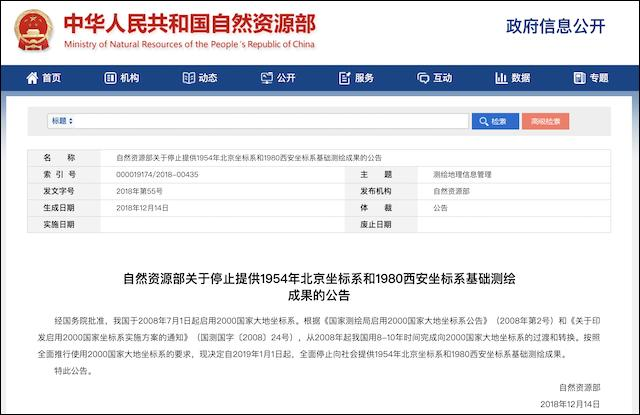自然资源部公告

6. 同别的坐标系之间的转换

在实际运用的过程中，往往会涉及到北京54坐标系同别的坐标系之间的转换，这一点，可以使用水经注万能地图下载器，在导出的时候可以选择北京54等各种坐标系，如下图所示。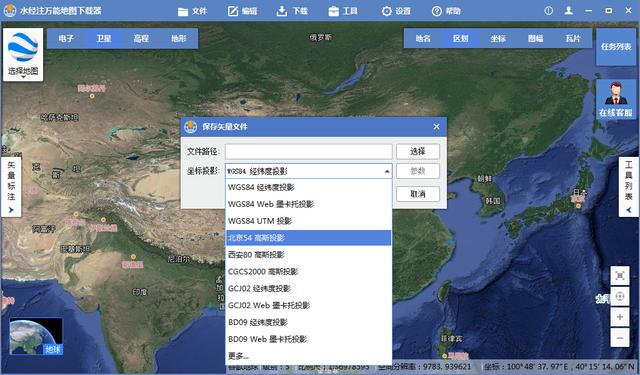坐标转换

7. 结语

以上就是北京54的详细说明，主要包括了坐标历史、椭球参数、坐标特点、坐标局限性和同别的坐标系之间的转换等功能。

展开全文• 基于MATLAB的WGS84坐标北京54坐标转换.pdf
• 1.椭球体、基准面及地图投影GIS中的坐标系定义是GIS系统的基础，正确定义GIS系统的坐标系非常重要。GIS中的坐标系定义由基准面和地图投影两组参数确定，而基准面的定义则由特定椭球体及其对应的转换参数确定，因此欲...
• 北京54 WGS84 90 80 高斯等坐标系之间的相互转换
• //坐标正算 lbxy(double l, double b, double *x, double *y, int l0) { double sa,sb,sep,sn,sy2,st,sm,sx,hb; double xx,yy,hd,sd; //判断值的范围 if (l > 360 || l < 0 || b > 360 || b < 0) { ...
• WGS84与北京54坐标转换研究，国际与国内主要应用坐标系统的转换研究
• 高斯坐标转换为北京54坐标系c代码，北京54坐标系，克拉索夫椭球...